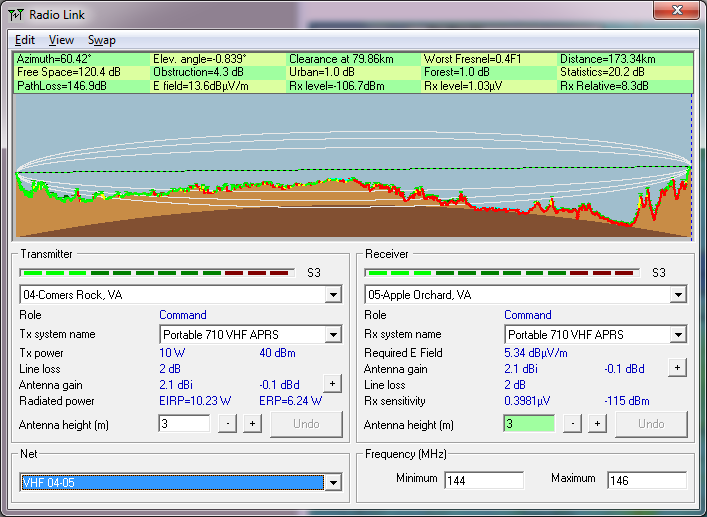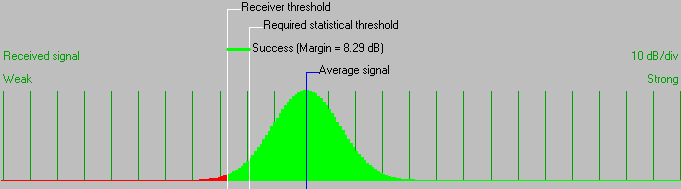• Distance between 04-Comers Rock, VA and 05-Apple Orchard, VA is 173.3 km (107.7 miles)
• True North Azimuth = 60.42°, Magnetic North Azimuth = 67.91°, Elevation angle = -0.8391°
• Terrain elevation variation is 981.5 m
• Propagation mode is line-of-sight, minimum clearance 0.4F1 at 79.9km
• Average frequency is 145.000 MHz
• Free Space = 120.4 dB, Obstruction = 4.3 dB, Urban = 1.0 dB, Forest = 1.0 dB, Statistics = 20.2 dB
• Total propagation loss is 146.9 dB (interference mode (optimistic))
• System gain from 04-Comers Rock, VA to 05-Apple Orchard, VA is 155.2 dB
• System gain from 05-Apple Orchard, VA to 04-Comers Rock, VA is 155.2 dB
• Worst reception is 8.3 dB over the required signal to meet 95.000% of situations